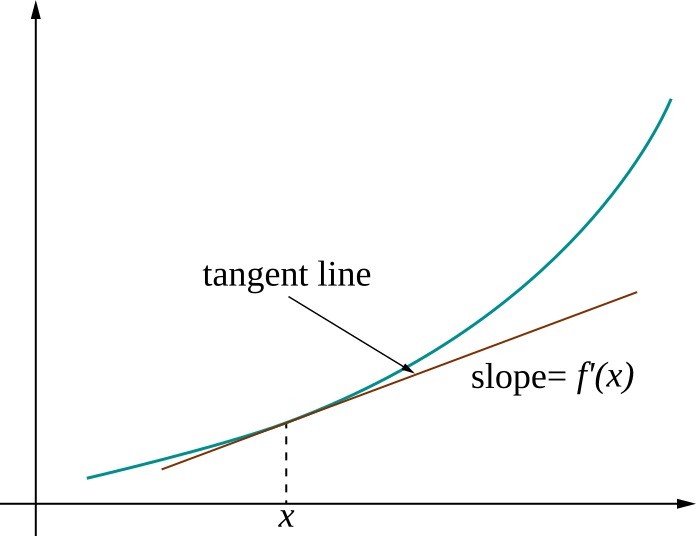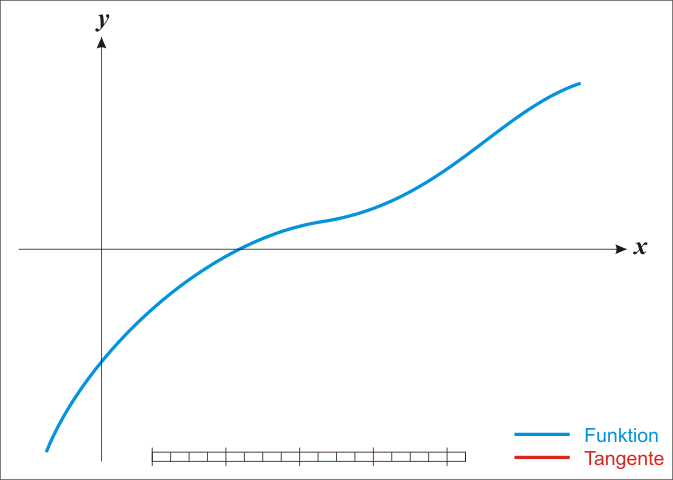# A review of vector calculus and concepts in optimization

## Instructions:

Use the left and right arrow keys to navigate the presentation forward and backward respectively. You can also use the arrows at the bottom right of the screen to navigate with a mouse.

FAIR USE ACT DISCLAIMER:
This site is for educational purposes only. This website may contain copyrighted material, the use of which has not been specifically authorized by the copyright holders. The material is made available on this website as a way to advance teaching, and copyright-protected materials are used to the extent necessary to make this class function in a distance learning environment. The Fair Use Copyright Disclaimer is under section 107 of the Copyright Act of 1976, allowance is made for “fair use” for purposes such as criticism, comment, news reporting, teaching, scholarship, education and research.

## Outline

• The following topics will be covered in this lecture:
• Concepts in analytical and numerical differentiation
• Newton’s method in one variable
• Tangent vectors, tangent spaces and vector fields
• The Jacobian, the inverse function theorem and Newton’s method in multiple variables
• Gradients, Hessians, and concepts in optimization

### Concepts in analytical differentiationCourtesy of Pbroks13, CC BY-SA 3.0, via Wikimedia Commons

• The derivative represents the slope of a tangent line to a curve.
• In the figure to the left, we see the function $$f$$ represented by the blue curve.
• The derivative $$f'(x)$$ at a given point gives the infinitesimal rate of change at that point with respect to small changes in $$x$$, denoted $$\delta_x$$.
• Suppose we have a point $$x_0$$, a nearby point that differs by only a small amount in $$x$$ $x_1 = x_0+\delta_{x_1},$
• The function $f(x_1) \approx f(x_0) + f'(x_0)\delta_{x_1}$ is what is known as the tangent line approximation to the function $$f$$.
• Such an approximation exists when $$f$$ is sufficiently smooth and is accurate when $$\delta_{x_1}$$ is small, so that the difference of $$x_1$$ from the fixed value $$x_0$$ is small.
• We can see graphically how the approximation becomes worse as we take $$\delta_{x_1}$$ too large.

### Concepts in analytical differentiation

• More generally, the tangent line approximation is one kind of general Taylor approximation.

• Suppose we have a point $$x_0$$ fixed, and define $$x_1$$ as a small perturbation $x_1 = x_0+\delta_{x_1},$

• If a function $$f$$ has $$k$$ continuous derivatives we can write $f(x_1) = f(x_0) + f'(x_0)\delta_{x_1} + \frac{f''(x_0)}{2!}\delta_{x_1}^2 + \cdots + \frac{f^{(k)}(x_0)}{k!} \delta_{x_1}^k + \mathcal{O}\left(\delta_{x_1}^{k+1}\right)$

• The $$\mathcal{O}\left(\delta_{x_1}^{k+1}\right)$$ refers to terms in the remainder, that grows or shrinks like the size of the perturbation to the power $$k+1$$.

• This is why this approximation works well when $$\delta_{x_1}$$ is a small perturbation.
• Another important practical example of using this Taylor approximation, when the function $$f$$ has two continuous derivatives, is $f(x_0 + \delta_{x_1}) \approx f(x_0) + f'(x_0)\delta_{x_1} + f''(x_0) \frac{\delta_{x_1}^2}{2}$ which will be used shortly for obtaining solutions to several kinds of equations.

• Particularly, this is strongly related to our second derivative test from univariate calculus.

## An approach to numerical derivation

• At the moment, we consider how Taylor's expansion can be used at first order again to approximate the derivative.

• Recall, we write

\begin{align} f(x_1) &= f(x_0) + f'(x_0) \delta_{x_1} + \mathcal{O}\left( \delta_{x_1}^2\right) \\ \Leftrightarrow \frac{f(x_1) - f(x_0)}{ \delta_{x_1}} &= f'(x_0) + \mathcal{O}\left( \delta_{x_1}\right) \end{align}

• This says that for a small value of $$\delta_{x_1}$$, we can obtain the numerical approximation of $$f'(x_0)$$ proportional to the accuracy of the largest decimal place of $$\delta_{x_1}$$ by the difference on the left hand side.

• This gives a forward finite difference equation approximation to the derivative.

• We can similarly define a backward finite difference equation with $$\pmb{x}_1 := \pmb{x}_0 -\pmb{\delta}_{\pmb{x}_1}$$.

• In each case, we use the perturbation to parameterize the tangent-line approximation.

## Newton's method in one variable

• We have seen earlier the basic linear inverse problem,

\begin{align} \mathbf{A}\pmb{x} = \pmb{b} \end{align} where $$\pmb{b}$$ is an observed quantity and $$\pmb{x}$$ are the unknown variables related to $$\pmb{b}$$ by the relationships in $$\mathbf{A}$$.

• We observed that a unique solution exists when all the relationships expressed by the columns are unique, corresponding to all non-zero eigenvalues.
• A similar problem exists when the relationship between $$\pmb{x}$$ and $$\pmb{b}$$ is non-linear, but we still wish to find some such $$\pmb{x}$$.

Nonlinear inverse problem (scalar case)
Suppose we know the nonlinear, scalar function $$f$$ that gives a relationship \begin{align} f(x^\ast) = b \end{align} for an observed $$b$$ but an unknown $$x^\ast$$. Finding a value of $$x^\ast$$ that satisfies $$f(x^\ast)=b$$ is known as a nonlinear inverse problem.
• Define a function \begin{align} \tilde{f}(x) = f(x)-b. \end{align}

• Thus solving the nonlinear inverse problem in one variable is equivalent to finding the appropriate $$x^\ast$$ for which \begin{align} \tilde{f}(x^\ast)= 0 . \end{align}

• Finding a zero of a function, or root finding, is thus equivalent to a nonlinear inverse problem.

• The Newton-Raphson method is one classical approach which has inspired many modern techniques.

### Newton's method in one variable

• We are searching for the point $$x^\ast\in \mathbb{R}$$ for which the modified equation $$\tilde{f}\left(x^\ast\right) = 0$$, and we suppose we have a good initial guess $$x_0$$.
• We define the tangent approximation as, $t(\delta_x) = \tilde{f}(x_0) + \tilde{f}'(x_0) \delta_x$ for some small perturbation value of $$\delta_x$$.
• Recall, $$\tilde{f}'(x_0)$$ refers to the value of the derivative of $$\tilde{f}$$ at the point $$x_0$$ – suppose this value is nonzero.
• In this case, we will examine where the tangent line intersects zero to find a better approximation of $$x^\ast$$.
• Suppose that for $$\delta_{x_0}$$ we have $\begin{matrix} t(\delta_{x_0}) = 0 & \Leftrightarrow & 0= \tilde{f}(x_0) + \tilde{f}'(x_0) \delta_{x_0} & \Leftrightarrow &\delta_{x_0} = \frac{-\tilde{f}(x_0)}{\tilde{f}'(x_0)} \end{matrix}$
• The above solution makes sense as long as $$f'(x_0)$$ is not equal to zero;
• if not, this says that the tangent line intersects zero at $$x_1 = x_0 + \delta_{x_0}$$, giving a new approximation of $$x^\ast$$.Courtesy of Ralf Pfeifer, CC BY-SA 3.0, via Wikimedia Commons

• The process of recursively solving for a better approximation of $$x^\ast$$ terminates when we reach a certain tolerated level of error in the solution or the process times out, failing to converge.
• This method has a direct analog in multiple variables, for which we will need to extend our notion of the derivative and Taylor’s theorem to multiple dimensions.

### Newton's method – example

• As a quick example, let's consider the Newton algorithm built-in to Scipy.

• Scipy is another standard library like Numpy, but which contains various scientific methods and solvers rather than general linear algebra.
• Specifically, we will import the built-in newton function from the optimize sub-module of scipy.

from scipy.optimize import newton

• In the following, we define the cubic function $$f(x):=x^3$$, but we are interested in the value $$x^\ast$$ for which $$f\left(x^\ast\right)=1$$

• The augmented function $$\tilde{f}(x):= x^3 - 1$$ defines the root-finding problem from the nonlinear inverse problem:
def f(x): return (x**3 - 1)

• The newton function can be supplied an analytical derivative, if this can be computed, to improve the accuracy versus, e.g., a finite-differences approximation.

• In the below, we supply this as a simple lambda function in the arguments of newton:
root = newton(f, 1.5, fprime=lambda x: 3 * x**2)
root

1.0


## Tangent vectors

• To expand our discussion to multiple variables, we will review some fundamental concepts of vector calculus.

• Suppose we have a vector valued function, with a single argument:

\begin{align} \pmb{x}:&\mathbb{R} \rightarrow \mathbb{R}^{N};\\ \pmb{x}(t) :=& \begin{pmatrix} x_1(t) & \cdots & x_{N}(t)\end{pmatrix}^\top; \end{align}

• prototypically, we will think of $$\pmb{x}(t)$$ as a curve in state-space, with its position at each time $$t\in\mathbb{R}$$ defined by the equation above.
Tangent vector
Suppose $$\pmb{x}(t)$$ is defined as above and that each of the component functions $$x_i(t)$$ are differentiable. The tangent vector to the state trajectory $$\pmb{x}$$ is defined as $\vec{x}:= \frac{\mathrm{d}}{\mathrm{d}t} \pmb{x}:= \begin{pmatrix}\frac{\mathrm{d}}{\mathrm{d}t} x_1(t) & \cdots & \frac{\mathrm{d}}{\mathrm{d}t} x_{N}(t)\end{pmatrix}^\top$
• In the above, the interpretation of the derivative defining a tangent line is extended into multiple variables;

• in this case, the tangent line is embedded in a higher-dimensional space of multiple variables.

### Tangent spaces

• An important extension of the tangent vector is the notion of the tangent space;
• this can be defined in terms of all differential perturbations generated at a point:
Tangent spaces
Let $$\pmb{x}\in\mathbb{R}^{N}$$ and $$\gamma(t)$$ be an arbitrary differentiable curve $$\pmb{\gamma}:\mathbb{R}\rightarrow \mathbb{R}^{N}$$ such that $$\pmb{\gamma}(0)= \pmb{x}$$ with a tangent vector defined as $$\vec{\gamma}(0):= \frac{\mathrm{d}}{\mathrm{d}t}|_0 \pmb{\gamma}$$. The tangent space at $$T_{\pmb{x}}$$ is defined by the linear span of all tangent vectors as such through $$\pmb{x}$$.
• In the above, we consider only the simplest definition of the tangent space;
• in this case the tangent space, $$T_{\pmb{x}} \equiv \mathbb{R}^{N}$$, is simply the space of all perturbations to the point $$\pmb{x}$$.
• However, this idea is extended into far greater generality: